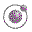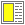Name binding, Recursion, Iteration, and Continuations - slide 38 : 42

The capturing of continuations
Scheme provides a primitive that captures a continuation of an expression E in a context C

The primitive is called call-with-current-continuation, or call/cc as a short alias

call/cc takes a parameter, which is a function of one parameter.

The parameter of the function is bound to the continuation, and the body of the function is E

 Context C and the capturing `(+ 5 (call/cc (lambda (e) (* 4 3)) ))` `(cons 1 (cons 2 (cons 3 (call/cc (lambda (e) '()) ))))` ```(define x 5) (if (= 0 x) 'undefined (remainder (* (call/cc (lambda (e) (+ x 1)) ) (- x 1)) x))```